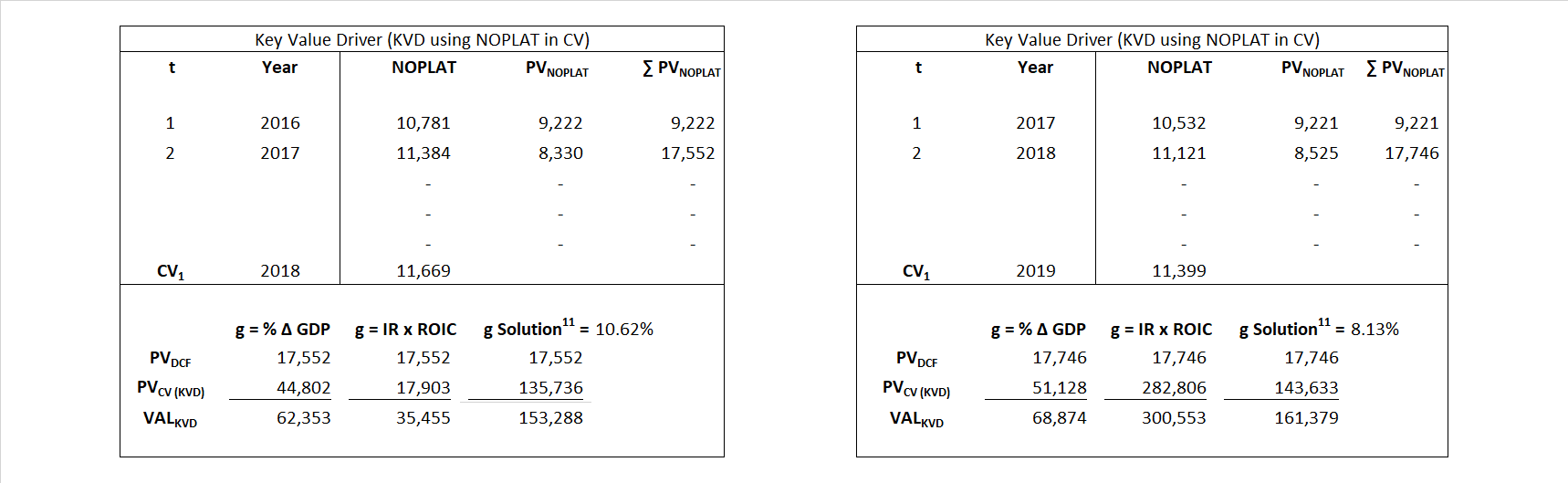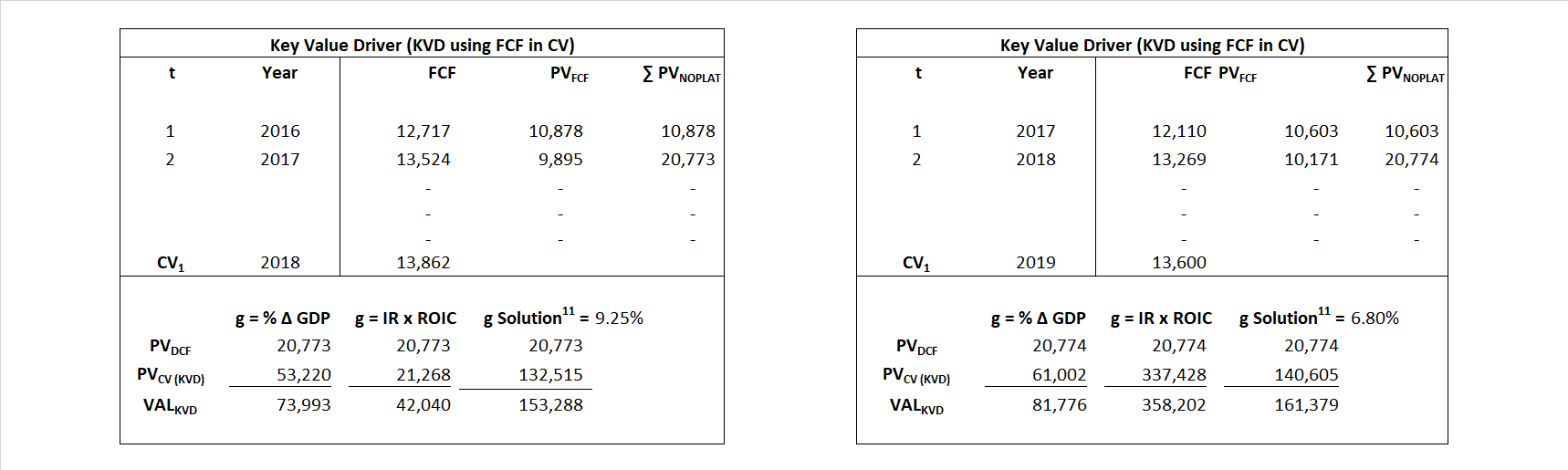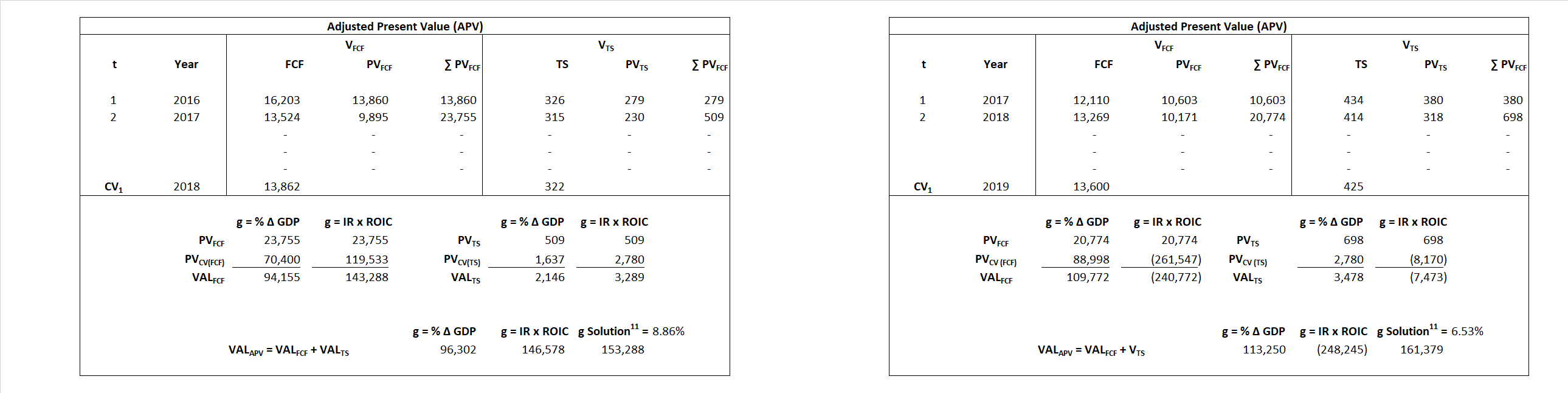# Oracle

## Analyst Listing

The following analysts provide coverage for the subject firm as of May 2016:

 Broker Analyst Analyst Email Stifel Nicolaus Brad R. Reback rebackb@stifel.com Pacific Crest Securities-KBCM Brendan Barnicle bbarnicle@pacific-crest.com Drexel Hamilton Brian J. White bwhite@drexelhamilton.com Oppenheimer Brian Schwartz brian.schwartz@opco.com Atlantic Equities Christopher Hickey c.hickey@atlantic-equities.com Nomura Research Frederick Grieb frederick.grieb@nomura.com DA Davidson Jack Andrews jandrews@dadco.com William Blair Jason Ader jader@williamblair.com BTIG Joel P. Fishbein jfishbein@btig.com Jefferies John DiFucci jdifucci@jefferies.com SunTrust Robinson Humphrey John Rizzuto john.rizzuto@suntrust.com Deutsche Bank Research Karl Keirstead karl.keirstead@db.com Daiwa Securities Co. Ltd. Kazuya Nishimura kazuya.nishimura@us.daiwacm.com BMO Capital Markets Keith Bachman keith.bachman@bmo.com Evercore ISI Kirk Materne kirk.materne@evercoreisi.com Bernstein Research Mark L. Moerdler mark.moerdler@bernstein.com Northland Securities Marshall Senk msenk@northlandcapitalmarkets.com Raymond James Michael Turits michael.turits@raymondjames.com JMP Securities Patrick Walravens pwalravens@jmpsecurities.com Credit Suisse Philip Winslow philip.winslow@credit-suisse.com Canaccord Genuity Richard Davis Jr. rdavis@canaccordgenuity.com Societe Generale Richard Nguyen richard.nguyen@sgcib.com Wunderlich Securities Rob Breza robbreza@wundernet.com RBC Capital Markets Ross MacMillan ross.macMillan@rbccm.com Wedbush Securities Steve Koenig steve.koenig@wedbush.com

## Primary Input Data## Derived Input Data

### Equational Form

Net Operating Profit Less Adjusted Taxes NOPLAT   9,288   8,586$NOPLAT\, =\, EBIT\, x\, (1 \,-\, Avg \,\,Tax\,\, Rate\,\, on\,\, EBIT)$
Free Cash Flow FCF  11,701  12,279$FCF\,=NOPLAT\,+\,Non-Cash\,Expenses-\Delta NWC\,-\,NCS$
Tax Shield TS  285  374$TS\,=\,Interest\,\,Paid\,\,x\,\, Avg \,\,Tax\,\,Rate\,\, on\,\, Pre-Tax\,\, Income$
Invested Capital IC  90,662  108,033$IC\,=\,Fixed\,\,Operating\,\,Assets\,\,+\,\,Net\,\, Working\,\, Capital$
Return on Invested Capital ROIC 10.24% 7.95%$ROIC\,=\,\frac { NOPLAT }{ IC }$
Net Investment NetInv  7,635  19,663$NetInv\,=\,{ {IC}_{1}}-{{IC}_{0}}+Depreciation$
Investment Rate IR 82.21% 229.01%$IR\,=\,\frac {NetInv}{NOPLAT}$
Weighted Average Cost of Capital WACCMarket 16.91% 14.21%$WACC\,=\,\frac { E }{ V } { R }_{ E }\,+\,\frac { P }{ V } { R }_{ P }\,+\,\frac { D }{ V } { R }_{ D }\left( 1- Avg\,\, Tax\,\,Rate\,\,on\,\,Pre-Tax\,\,Income \right)$
WACCBook  8.88% 8.04%
Enterprise value EVMarket  153,288  161,379$EV\,=\,Market\,\,Cap\,\,Equity\,+\,\,Long\,\,Term\,\,Debt\,-\,Cash$
EVBook  145,338  156,801
EV/EBIT Multiple$\frac{EV_{Market}}{EBIT}$  10.73  12.22$EV/EBIT\,=\,\frac { EV}{ EBIT}$
Long-Run Growth g = IR x ROIC
8.42%   18.20% Long-run growth rates of the income variable  are used in the Continuing Value portion of the valuation models.
g = %$\Delta$ GDP   2.50%   2.50%

## Valuation Model Outcomes

The outcomes presented in this study are the result of original input data, derived data, and synthesized inputs and, depending on the equational form of any particular valuation model, may result in irrelevant or implausible results.  For example, in the event WACC < g, the value of this term, often found in the denominator of an equation’s continuation value term, will be expressly negative and may result in a negative overall valuation for the firm.  In the event of a WACC < g relation, the model form as applied to the subject firm offers an irrelevant outcome.

### Equational form

Key Value Driver (NOPLAT) KVD (NOPLAT)${ Value }_{ DCF/KVD }=\sum { \frac { NOPLAT_{ t } }{ { \left( 1+WACC \right) }^{ t } } +\frac { \frac { { NOPLAT }_{ 1 }\left( 1-\frac { g }{ ROIC } \right) }{ WACC-g } }{ { \left( 1+WACC \right) }^{ t } } }$Key Value Driver (FCF) KVD (FCF)${ Value }_{ DCF/KVD }=\sum { \frac { FCF_{ t } }{ { \left( 1+WACC \right) }^{ t } } +\frac { \frac { { NOPLAT }_{ 1 }\left( 1-\frac { g }{ ROIC } \right) }{ WACC-g } }{ { \left( 1+WACC \right) }^{ t } } }$Free Cash Flow FCF${ Value }_{ DCF/FCF }=\sum { \frac { FCF_{ t } }{ { \left( 1+WACC \right) }^{ t } } +\frac { \frac { { FCF }_{ 1 }}{ WACC-g } }{ { \left( 1+WACC \right) }^{ t } } }$Economic Profit ECON π${ Value }_{ { ECON\pi } }= I{ C }_{ 0 }+\sum { \frac { { IC }_{ t-1 }(ROI{ C }_{t}-WAC{C}_{t}) }{ { \left( 1+WACC \right) }^{ t } }+ \frac {\frac { I{C}_{0}\ x\ (ROI{C}_{1}\ -\ WAC{C}_{1}) }{ WACC-g } }{ { \left( 1+WACC \right) }^{ t } } }$Adjusted Present Value APV${ Value }_{ APV }=\sum { \frac { FCF_{ t } }{ { \left( 1+{ k }_{ u } \right) }^{ t } } +\frac { \frac { { FCF }_{ 1 }}{ { k }_{ u }-g } }{ { \left( 1+{ k }_{ u } \right) }^{ t } } } +\sum { \frac { { TS }_{ t } }{ { \left( 1+{ k }_{ tax } \right) }^{ t } } +\frac { \frac { { TS }_{ 1 }}{ { k }_{ tax }-g } }{ { \left( 1+{ k }_{ tax } \right) }^{ t } } }$Forward Market Multiple FMM${ Value }_{ DCF/FMM}=\sum { \frac { FCF_{ t } }{ { \left( 1+WACC \right) }^{ t } } +\frac { { EBIT }_{ 1 }\,{x}\,{FMM}}{ { \left( 1+WACC \right) }^{ t } } }{\,\,\,; \,\,FMM\,=\,\frac{{EV}_{t=0}}{{EBIT}_{t=0}}}$